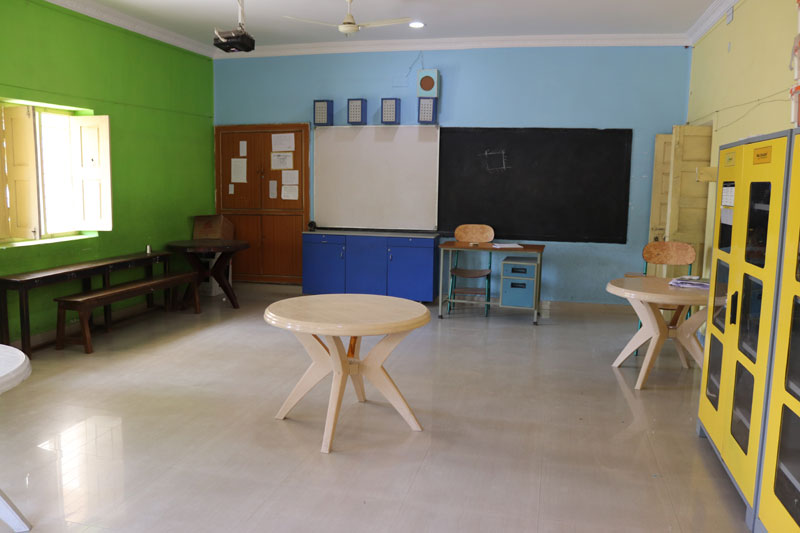## Maths LabThe mathematics laboratory is a place rich in manipulated material, to which children have

mathematical puzzles and become involved in other activities.

An integral part of the mathematics laboratory is the manipulation of the objects through

manipulating concrete material, the child is better able to bridge the gap between the real and the

abstract previous implements.

The use of mathematics lab helps to integrate theory and practical in mathematics teaching and

learning. Display mathematical information avenue for experimentation through practical work.

Pool of storage of mathematical for easy access.

Based on the advantages of mathematics laboratory it is expected that teaching and learning

mathematics with laboratory may helps to reduce the abstract nature of the subject and draw the

students to follow.

Students Benefits

Improving confidence in learning by doing and practicing.

Play way learning improves interest and attention span.